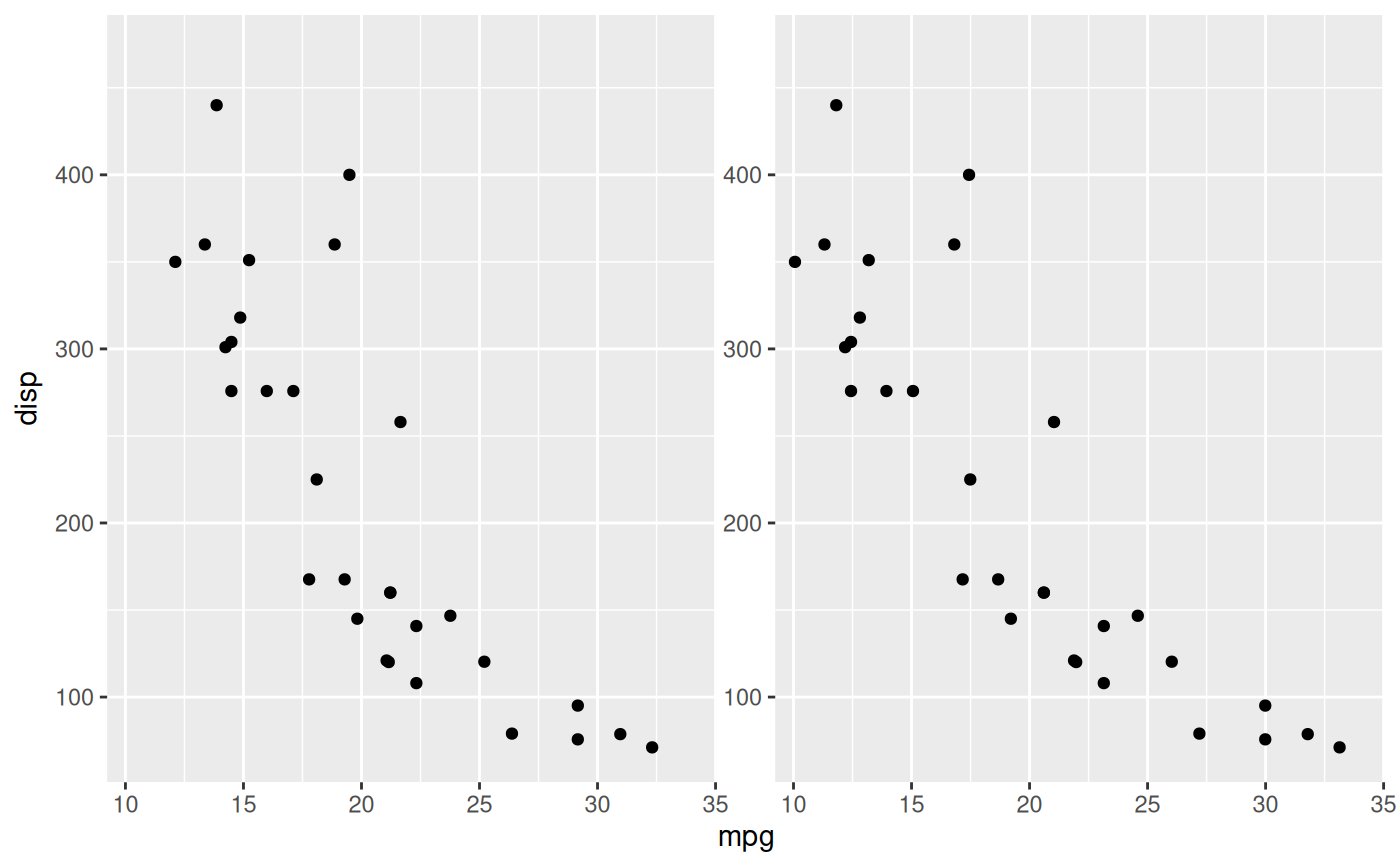These scales serve to scale the depth aesthetic when creating stereographic plots. The range specifies the relative distance between the points and the paper plane in relation to the distance between the eyes and the paper plane i.e. a range of c(-0.5, 0.5) would put the highest values midways between the eyes and the image plane and the lowest values the same distance behind the image plane. To ensure a nice viewing experience these values should not exceed ~0.3 as it would get hard for the eyes to consolidate the two pictures.

scale_depth(..., range = c(0, 0.3))

scale_depth_continuous(..., range = c(0, 0.3))

scale_depth_discrete(..., range = c(0, 0.3))

## Arguments

...

arguments passed on to continuous_scale or discrete_scale

range

The relative range as related to the distance between the eyes and the paper plane.

## Examples

ggplot(mtcars) +
geom_point(aes(mpg, disp, depth = cyl)) +
scale_depth(range = c(-0.1, 0.25)) +
facet_stereo()
#> Warning: Ignoring unknown aesthetics: depth